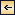Dynamics of Ocean Currents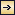### Wind Currents

The Stress of the Wind. From laboratory results dealing with flow over smooth and rough surfaces, one may expect that the stress can be expressed by means of equation (XIII, 59), which gives the stress over a smooth surface, or equation (XIII, 63), which gives the stress over a rough surface. The density, ρ, which enters in the formulae is then the density of the air. In order to determine whether the sea surface can be considered smooth or rough and, if it is rough, to determine the value of the roughness length, zo, simultaneous measurements of wind velocities must be made at three or more levels above the sea surface. From such measurements conducted by Wüst (1920), Rossby and Montgomery (1935) concluded that at moderate wind velocities the sea surface has the character of a rough surface with roughness length 0.6 cm. From a general theory of the wind profile in the lower part of the atmosphere, Rossby (1936b) later developed formulae that permit computation of zo from the angle between the surface wind and the gradient wind (corresponding to no friction) or from the ratio between surface-wind velocity and gradient-wind velocity. When applying these formulae to observed values from the ocean, Rossby found that at moderate and strong winds the roughness length is independent of the wind velocity and equal to

490
0.6 cm. With this value of zo and with ko = 0.4, one obtains from formula (XIII, 63)[Equation]
where ρ′ is the density of the air and W15 is the wind velocity 15 m above the sea surface.

A similar expression was derived by Ekman as early as 1905 through study of the slope of the sea surface during a storm in the Baltic in 1872. A. Colding had found that the lines of equal water level were directed nearly perpendicular to the direction of the wind. The sea-level values were obtained from records at stations on the coast, and thus represent the values of the physical sea level. The relation between wind velocity, W, depth, d, and slope, i, could be expressed by the equation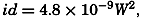[Equation]
where all quantities are expressed in c.g.s. units but where the numerical factor is not nondimensional. Ekman (1905) entered this expression in equation (XIII, 72), putting gρ = 1000. In this case, i could be put equal to is., because, in the shallow Baltic, the first term is insignificant. Ekman shows that, on certain assumptions, τd, the stress exerted against the bottom, must lie between 0 and 1/3τa.

In the case examined by Colding, Ekman assumes that τa + τd = 3/2τa, and obtains, therefore,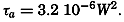[Equation]
Introducing the density of the air, ρ′ = 1.25 × 10−3, one obtains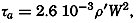[Equation]
where the numerical constant is nondimensional. The equation is probably applicable to wind velocities of about 20 m /sec at an altitude of 15 m above the sea surface.

Palmén and Laurila (1938) have made a similar study of the effect of the wind on the sea level in the Gulf of Bothnia during a storm in October, 1936, and obtained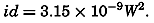[Equation]
The formula is valid between wind velocities of 10 m/sec and 26 m/sec at an altitude of about 10 m above the sea surface. The average depth was taken as 50 m, but this value is somewhat uncertain. They assume τd = 0 and obtain, with ρ′ = 1.3 × 10−3,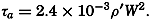[Equation]

These results, although uncertain, confirm the conclusion that at moderate and strong winds the stress of the wind is proportional to

491
the square of the wind velocity, the factor of proportionality being about 2.6 × 10−3ρ′. If equation (XIII, 74) is considered valid, the implication is that at moderate and strong wind the roughness length is independent of the wind velocity. This conclusion appears surprising, in view of the fact that the height of the waves increases with increasing wind velocity, but it must be borne in mind that the waves travel with the wind. The above conclusion, therefore, implies that the speed at which the waves travel stands in such a relation to the wind velocity that the effective roughness of the sea surface remains constant.

At low wind velocities, somewhat different conditions appear to prevail. From a study of simultaneous wind measurements at different heights above the sea, Rossby (1936b) concludes that at low wind velocities the sea surface has the character of a smooth surface, because the wind profiles indicate the existence of a laminar sublayer. At low wind velocities the stress should therefore be computed from equation (XIII, 59), which gives values that are about one third of those obtained on the assumption that the surface is rough.

Table 67 shows the values of the stress corresponding to a smooth surface or a rough surface characterized by z0 = 0.6 cm.

STRESS OF THE WIND (G/CM/SEC2) CORRESPONDING TO STATED WIND VELOCITIES (IN M/SEC) AT A HEIGHT OF 15 M AND ASSUMING THE SURFACE TO BE SMOOTH OR ROUGH (ROUGHNESS LENGTH, 0.6 CM)
Surface Wind velocity in m/sec at 15 m
2 4 6 8 10 12 14 16 18
“Smooth” 0.04 0.16 0.34 (0.58)
“Rough” (0.11) (0.45) (1.01) 1.81 2.83 4.09 5.56 7.25 9.20

It is evident that, if the sea surface can be considered smooth at wind velocities below 6 to 7 m/sec, the stress of wind velocities below this limit is of little significance, but the above conclusions need to be confirmed by means of many more data. The problem of the stress of the wind still deserves great attention, and it is particularly desirable to obtain more measurements of wind profiles, because results in fluid mechanics can be successfully applied in the study of such profiles.

The theoretical equations for the relations between stress and wind velocity are valid only if the stability of the air is nearly indifferent. Under stable or unstable conditions the wind profiles will be different, and the relationships between wind velocity and stress will be altered (Sverdrup, 1939b). A study of wind profiles at different stabilities of the air is therefore of great interest.

492

Wind Currents in Homogeneous Water. In 1902, V. Walfrid Ekman published his classical paper on wind currents in which, for the first time, the effect both of the deflecting force of the earth's rotation and of the eddy viscosity was taken into account. Ekman undertook the mathematical analysis at the suggestion of Fridtjof Nansen, who, during the drift of the Fram across the Polar Sea in 1893–1896, had observed that the ice drift deviated 20° to 40° to the right of the wind, and had attributed this deviation to the effect of the earth's rotation. Nansen further reasoned that the direction of the motion of each water layer must deviate to the right of the direction of movement of the overlying water layer in a similar manner, because it is swept on by this layer much like the ice, which covers the surface, is swept on by the wind. Therefore, at some depth the current would run in a direction opposite to that of the surface flow. These conclusions of Nansen's were fully confirmed by the mathematical treatment.

In homogeneous water the equations of motion of a steady state take the form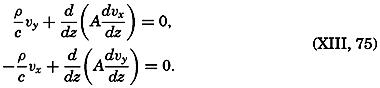[Equation]
To these equations must be added the equation of continuity and the boundary conditions.

Assuming that the eddy viscosity is independent of depth, one can integrate the equations directly. Writing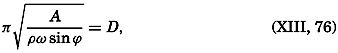[Equation]
one obtains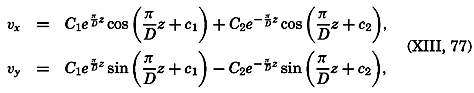[Equation]
where C1, C2, c1, and c2 are constants that must be determined by means of the boundary conditions.

In this form the result is applicable to conditions in the Northern Hemisphere only, because in the Southern Hemisphere sin ϕ is negative, wherefore D is imaginary. In order to obtain a solution that is valid in the Southern Hemisphere, the direction of the position of the y axis must be reversed.

The solution takes the simplest form if the depth to the bottom is so great that one can assume no motion near the bottom, because then

493
C1 must be zero. Assuming, furthermore, that the stress of the wind, τa, is directed along the y axis, one has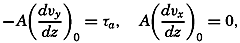[Equation]
and from these equations C2 and c2 can be determined. Calling the velocity at the surface ν0, one obtains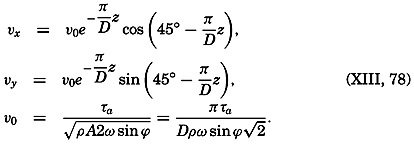[Equation]

Therefore, the wind current is directed 45° cum sole from the direction of the wind. The angle of deflection increases regularly with depth, so that at the depth z = D the current is directed opposite to the surface current. The velocity decreases regularly with increasing depth, and at z= D is equal to e−x times the surface velocity, or one twenty-third of the value at the surface. By far the more important velocities occur above the depth z = D, and Ekman has therefore called this depth “the depth of frictional resistance.” It should be observed, however, that according to this solution the velocity of the wind current never becomes zero, but approaches zero asymptotically and is practically zero below z = D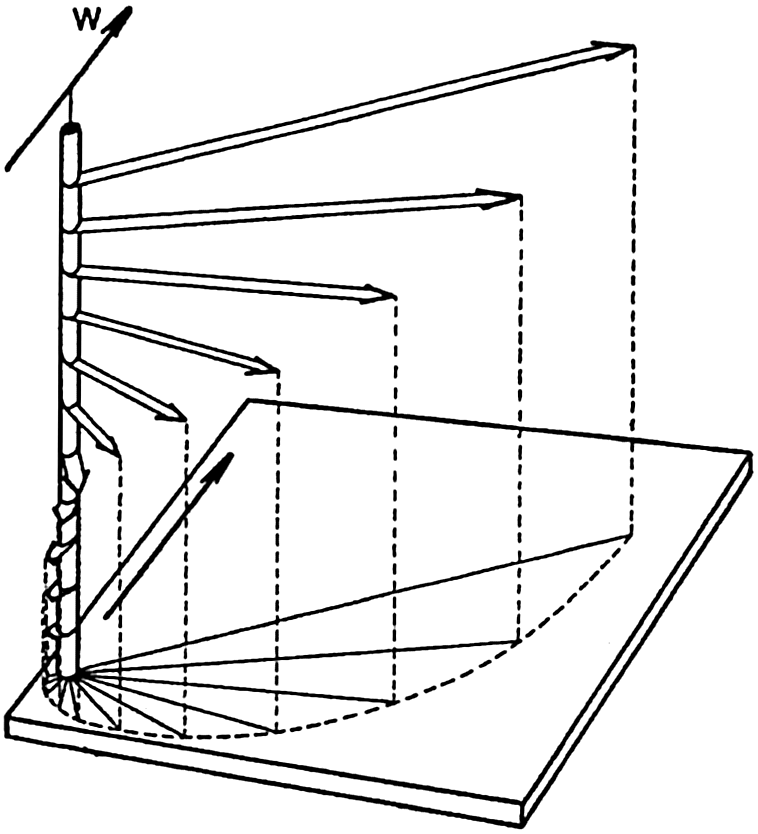#### Schematic representation of a wind current in deep water, showing the decrease in velocity and change of direction at regular intervals of depth (the Ekman spiral). W indicates direction of wind.

[Full Size]

A schematic representation of the pure wind current is given in fig. 121. The broad arrows represent the velocities at depths of equal intervals. Together they form a spiral staircase, the steps of which rapidly decrease in width as they proceed downward. Projected on a horizontal plane the end points of the vectors lie on a logarithmic spiral.

494

The average deflection of the wind current from the direction of the wind has been examined (Krümmel, 1911) and found to be about 45° cum sole from the wind direction, independent of latitude, in agreement with Ekman's theory.

The ratio between the velocity of the surface current, which Thorade has called the wind factor, depends upon the stress of the wind and upon the value of the eddy viscosity—that is, upon D. The stress of the wind is proportional to the square of the wind velocity, τa = 3.2 10−6W2 (p. 490), where the wind velocity, W, is measured in centimeters per second. Introducing this value into (XIII, 78), one obtains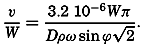[Equation]
On the basis of observations by Mohn and Nansen, Ekman derived the empirical relation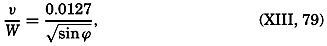[Equation]
from which follows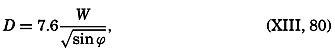[Equation]
a value that is in fair agreement with observed values of the upper homogeneous layer in the sea. This layer is interpreted as the layer which is stirred up by the wind. At wind velocities below 3 Beaufort (about 6 m/sec) the above formula, according to Thorade (1914), should be replaced by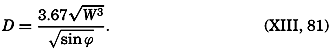[Equation]
The last two formulae give the depth of frictional resistance in meters for wind velocities in meters per second. When the wind velocity is below 4.3 m/sec, the latter formula gives smaller values of D than does Ekman's formula, perhaps because the stress of the wind is relatively smaller at low wind velocities (p. 491).

From equations (XIII, 76), (XIII, 80), and (XIII, 81), one obtains (see table 64)[Equation]
From these relations the following corresponding values are computed:

 Wind velocity (m/sec) 2 4 6 8 10 15 20 A (g/cm/sec) 8 65 (218) 375 430 970 1720

Rossby (1932) and Rossby and Montgomery (1935) have developed a new theory of the wind currents by introducing an eddy viscosity that

495
depends upon a mixing length which is small near the surface, increases to a maximum at a short distance below the surface (p. 479), and then decreases linearly to zero at the lower boundary of the wind-current layer. The variation with depth of the mixing length is assumed to be entirely determined by means of dimensionless numerical constants. The theory leads to a series of complicated expressions for the angle between surface current and stress, for the wind factor, and for the depth of the wind current. These quantities all depend both on latitude and on wind velocity. Rossby and Montgomery give their final results in the form of tables, according to which the deflection of the wind current in lat. 5° increases from 35° at a wind velocity of 5 m/sec to 43° at a wind of 20 m/sec, and in lat. 60° from 42° to 52.7°. In the same latitude the wind factors decrease from 0.0317 at a wind velocity of 5 m/sec to 0.0266 at a velocity of 20 m/sec, and from 0.0273 to 0.0228, respectively.

According to Ekman's theory the angle should remain constant at 45°, and the wind factor, according to the empirical results that he made use of, should be equal to 0.025 in lat. 15° and 0.0136 in lat. 60°. Rossby and Montgomery point out that the wind factor depends upon the depth at which the wind current is measured. Their theoretical values apply to the current at the very surface, but wind currents derived from ships' logs will apply to a depth of 2 or 3 m, depending upon the draft of the vessel. At this depth, their theory gives a wind factor in better agreement with Ekman's value, and they show that their theoretical conclusions are in fair agreement with empirical results. However, the introduction of an eddy viscosity that decreases to zero at a lower limit of the wind-stirred layer is justifiable only if no other currents are present. In the presence of other currents, such as tidal currents or currents related to distribution of mass, the eddy viscosity characteristic of the total motion must be introduced, and the variation of this eddy viscosity probably depends more upon the stability of the stratification than upon the geometric distance from the free surface. A theory of the wind currents must take this fact into account, but such a theory cannot be developed until more is known of the actual character of the turbulence. At present, Ekman's classical theory appears to give a satisfactory approximation, especially because no observations are as yet available by means of which the results of a refined theory can be tested.

So far, it has been assumed that the depth of the water is great compared to the depth of frictional resistance. Ekman has also examined the wind currents in shallow water and has determined the constants in equations (XIII, 77) by assuming that at the bottom the velocity is zero. This analysis leads to the result that in shallow water the deflection of the surface current is less than 45° and that the turning with depth is slower. In very shallow water the current flows nearly in the direction of the stress at all depths.

496

The assumption of an eddy viscosity that is independent of depth is not valid, however, if the water is shallow, because the eddy viscosity must be very small near the bottom (p. 480), regardless of the character of the current. The effect of a decrease of the eddy viscosity toward the bottom is generally that the angle between wind and current becomes greater at all depths and that the current velocities become greater. This effect is illustrated by measurements of wind currents on the North Shelf in lat. 76°35′ N, long. 138°24′ E (Sverdrup, 1929), where the depth to the bottom was 22 m. From these observations, Fjeldstad (1929) found that the eddy viscosity could be represented by the formula[Equation]
where the distance z from the bottom is in meters. Figure 122 shows the observed velocities and the corresponding velocities computed with Fjeldstad's equations and with Ekman's equations, assuming a constant value of A equal to 200.#### Wind current in shallow water assuming a constant eddy viscosity (dashed curve) or an eddy viscosity which decreases towards the bottom (full-drawn curve). Observed currents indicated by crosses.

[Full Size]

The question of the time needed for establishing the assumed stationary conditions has also been examined by Ekman (1905), who has made use of a solution given by Fredholm for the case of a wind that suddenly begins to blow with a velocity which later remains constant. It is found that the motion will asymptotically approach a steady state. At each depth the end points of the velocity vectors, when represented as a function of time, will describe a spiral around the end point of the final velocity vector, the period of oscillation being 12 pendulum hours, corresponding to the period of oscillation by inertia movement (p. 438). The average velocity over 24 hours will be practically stationary from the very beginning, but the oscillations around the mean motion may continue for several days and may appear as damped motion in the circle of inertia.

Wind Currents in Water in Which the Density Increases with Depth. In the equations of motion which govern the wind current, the density enters explicitly, and it might therefore be expected

497
that variations of density in a vertical direction would modify the results, but the variations of the density in the ocean are too small to be of importance in this respect. Indirectly the variations of density do greatly modify the wind current, however, by influencing the eddy viscosity of the water.

The rate at which a wind current penetrates toward greater depths will also depend upon the change of density with depth. Where an upper, nearly homogeneous layer of considerable thickness has already been developed by cooling from above and resulting vertical convection, the wind current will, in a short time, reach its normal state. Where a light surface layer has been developed because of heating or, in coastal areas, because of addition of fresh water, the wind current will first stir up the top layer and by mixing processes create a homogeneous top layer. When this layer is formed, the stability will be great at its lower boundary; there the eddy viscosity will be small and a further increase of the thickness of the homogeneous top layer will be effectively impeded, although the thickness may be much less than that of the layer within which normally a wind current should be developed. The further increase must be very slow, but no estimate can be given of the time required for the wind current to penetrate to the depths that it would have reached in homogeneous water. The gradual increase of the homogeneous top layer is shown schematically in fig. 123A.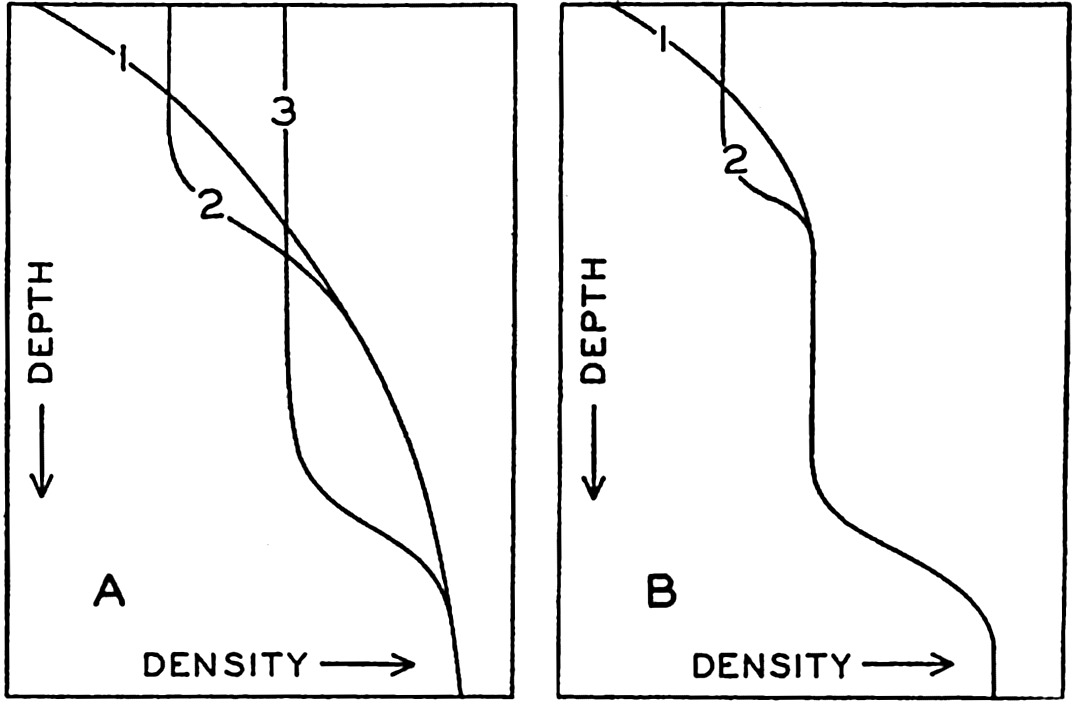#### Effect of wind in producing a homogeneous surface layer demonstrated by showing progressive stages of mixing.

[Full Size]

If the wind dies off, heating at the surface may again decrease the density near the surface, but as soon as the wind again starts to blow, a new homogeneous layer is formed near the surface, and consequently two sharp bends in the density curve may be present (fig. 123B).

498

If conclusions are to be drawn from the thickness of the upper homogeneous layer as to the depth to which wind currents penetrate, cases must be examined in which the wind has blown for a long time from the same direction and with nearly uniform velocity. In middle and high latitudes the wind current will reach its final state more rapidly in winter, when cooling takes place at the surface, than in summer, when heating takes place.

Transport and Energy Equations Applied to Wind Currents. The transport by wind currents across a vertical surface 1 cm wide is obtained by integration of the equations of motion, taking the boundary condition into account. In the open ocean, where the water is deep, the result is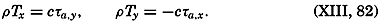[Equation]
Thus the transport is independent of the value of the eddy viscosity and is directed, in the Northern Hemisphere, 90° to the right of the wind, and in the Southern Hemisphere, 90° to the left of the wind. It is directly proportional to the stress of the wind and inversely proportional to the size of the latitude.

If in homogeneous water the kinetic energy remains constant, the energy equation (XIII, 68) is reduced to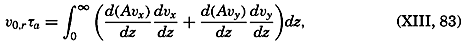[Equation]
where τa, as before, is the stress of the wind, and ν0,τ is the surface velocity in the direction of the wind. Thus, the power of the wind stress on the surface equals the dissipation.

In Ekman's solution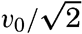[Equation], where ν0 is the velocity of the surface wind current, which deviates 45° from the direction of the wind. Because A is assumed to be constant, one obtains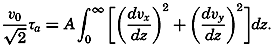[Equation]
The correctness of this equation can be verified by introducing the equations for νx, νy, and ν0 (XIII, 78).

In nonhomogeneous water the complete energy equation has to be considered. The power of the wind stress on the surface must equal the work performed against the pressure gradients and the loss of energy by dissipation due to friction. This is only another way of stating that the wind currents are modified in stratified water, but so far the extent of modification has not been examined.

One of the characteristics of the modifications can be demonstrated as follows. The boundary condition must always be fulfilled (XIII, 65); that is,

499
that is,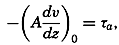[Equation]
where ν is the velocity in the direction of the tangential stress of the wind. In water in which the density varies with depth, the relative current generally will vary with depth, and therefore the above equation can be written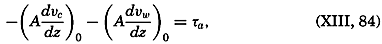[Equation]
where νc is the component of the relative current in the direction of the wind, and νw is the component of the wind current. If dvc/dz is negative—that is, if Vc decreases with depth—the wind current will be smaller than it is in homogeneous water. However, dVc/dz is usually so small that (Advc/dz) can be neglected.

Effect of Friction on Bottom Currents. A current flows along the bottom if the isobaric surfaces are inclined near the bottom, and in this case the effect of friction cannot be neglected. The motion must be zero at the bottom, and directly over the bottom the eddy viscosity must increase rapidly with the distance from the bottom (see p. 480).

The equations of motion can easily be integrated if one assumes that the eddy viscosity is constant and that near the bottom the slope of the isobaric surfaces is independent of the distance from the bottom. The latter assumption implies that in the absence of friction the “gradient velocity,” Vi = − gci, is independent of the distance from the bottom. Placing the center of the coordinate system at the bottom, the positive y axis in the direction of the slope of the isobaric surfaces, and the z axis positive upward, one obtains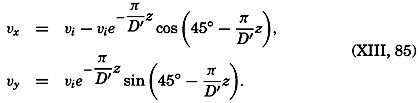[Equation]

This solution, which was given also by Ekman, corresponds exactly to the solution which represents the wind current, and can be represented in a similar manner (see fig. 121, p. 493). Above the bottom the current first turns to the right and its velocity increases until, above the depth D′, which corresponds to the depth of frictional resistance, it practically attains the constant velocity vi. Projected on a horizontal plane, the end points of the velocity vectors lie on a logarithmic spiral.

This solution gives a first orientation as to the effect of friction near the bottom, but it cannot be expected that the solution will give a

500
velocity distribution in good accord with actual conditions. The main reason for this statement is that the eddy viscosity cannot be expected to be constant from the bottom upwards, but must be nearly zero at the bottom, and in the lowest layers it probably increases linearly with the distance from the bottom. A theory of the probable variation of velocity with increasing distance from the bottom can be developed similar to Rossby's and Montgomery's theory (1935) of the layer of frictional resistance in the atmosphere, but so far such a theory has been of no interest because the necessary data for testing it are lacking. Actually, no observations exist that indicate the type of motion near the bottom, and the only statements which can be made with certainty are that, owing to the friction at the boundary, the flow near the bottom must have a component in the direction of the slope of the isobaric surfaces, and that the transport in the direction of the slope must be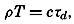[Equation]
where τd is the frictional stress at the bottom.

The above considerations are valid if the depth of the water is greater than the thickness of the layer of frictional resistance. In shallow water, modifications are encountered which have been discussed by Ekman on the assumption of a constant eddy viscosity.

Secondary Effect of Wind Toward Producing Currents. Upwelling. In the open ocean the total mass transport by wind is equal to a and is directed normal to the wind regardless of the depth to which the wind current reaches and regardless of the variation with depth of the eddy viscosity. This fact is of the greatest importance, because the transport of surface layers by wind plays a prominent part in the generation and maintenance of ocean currents. Boundary conditions and converging or diverging wind systems must, in certain regions, lead to an accumulation of light surface waters and, in other regions, to a rise of denser water from subsurface depths. Thus, the wind currents lead to an altered distribution of mass and, consequently, to an altered distribution of pressure that can exist only in the presence of relative currents. These processes will be illustrated by a few examples.

Consider in the Northern Hemisphere a coast line along which a wind blows in such a manner that the coast is on the right-hand side of an observer who looks in the direction of the wind (see fig. 124). At some distance from the coast the surface water will be transported to the right of the wind, but at the coast all motion must be parallel to the coast line. Consequently a convergence must be present off the coast leading to an accumulation of light water along the coast. This accumulation creates a relative field of pressure with which must be associated a current running parallel to the coast in the direction of the wind. Thus, the wind produces not only a pure wind current but also

501
a relative current that runs in the direction of the wind. It has not been possible as yet to examine theoretically the velocity distribution within this relative current, but it is a priori probable that this current becomes more and more prominent the longer the wind blows. As the current increases in speed, eddies will probably develop, and these eddies may limit the velocities which can be attained under any given circumstances. Also, it is probable that a vertical circulation will be present which will consume energy and tend to limit the velocities that can be reached.

Consider next a wind in the Northern Hemisphere which blows parallel to the coast, with the coast on the left-hand side. In this case the light surface water will be transported away from the coast and must, owing to the continuity of the system, be replaced near the coast by heavier subsurface water. This process is known as upwelling, and is a conspicuous phenomenon along the coasts of Morocco, Southwest Africa, California, and Peru (pp. 702 and 725). The upwelling also leads to changes in the distribution of mass, but now the denser, upwelled water accumulates along the coast and the light surface water is transported away from the coast. This distribution of mass again will give rise to a current that flows in the direction of the wind.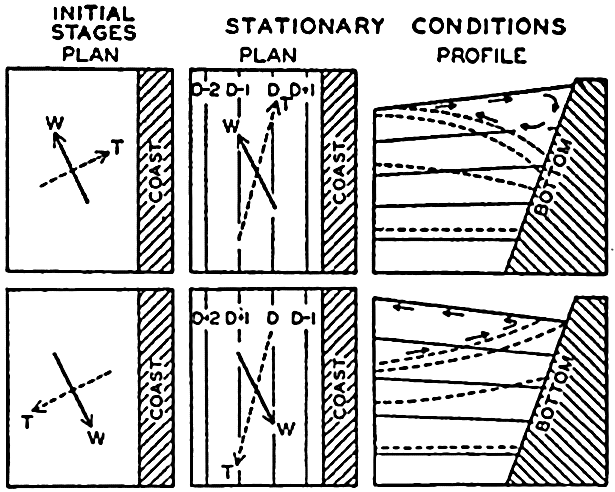#### Schematic representation of effect of wind towards producing currents parallel to a coast in the Northern Hemisphere and vertical circulation. W shows wind direction, and T, direction of transport. Contours of sea surface shown by lines marked D, D + 1. … Top figures show sinking near the coast; bottom figures show upwelling.

[Full Size]

The qualitative explanation of the upwelling was first suggested by Thorade (1909) and was developed by McEwen (1912). Recent investigations by Gunther (1936), Defant (1936b), Sverdrup (1930, 1938b), and Sverdrup and Fleming (1941) have added to the knowledge of the phenomenon, and have especially shown that water is drawn to the surface from depths not exceeding 200 to 300 m. Deep water does not rise to the surface, but an overturning of the upper layer takes place.

In spite of the added knowledge, it is as yet not possible to discuss quantitatively the process of upwelling or to predict theoretically the velocity and width of the coastal current that develops. It is probable that the tendency of the current is to break up in eddies, and that the forced vertical circulation limits the development of the current. Also, the wind that causes the upwelling does not, as a rule, blow with a steady velocity, and variations of the wind may greatly further the formation of eddies.

502

Figure 125 demonstrates the effect of winds from different directions on the currents off the coast of southern California in 1938. The charts show the geopotential topography of the sea surface relative to the 500-decibar surface, which in this case can be considered as nearly coinciding with a level surface. Arrows have been entered on the isolines, indicating that these are approximately stream lines of the surface currents.

In the absence of wind, one should expect a flow to the south or southeast, more or less parallel to the coast. In February, 1938 (fig. 125A), winds from the south or southwest had been blowing for some time. The light surface water had been carried toward the coast, and, consequently, a coastal current running north was present and was separated from the general flow to the south by a trough line, which probably represents a line of divergence. This inshore current to the north may not be an effect of the wind only, however, but may represent a countercurrent that develops when variable winds blow (p. 677).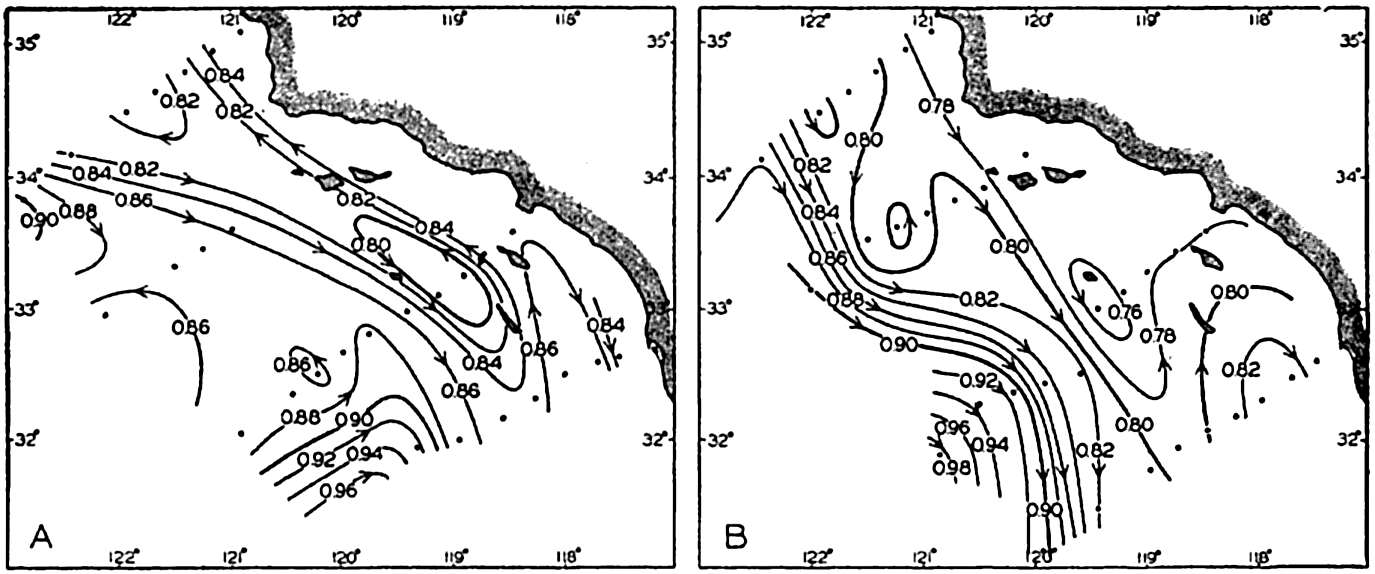#### (A) Geopotential topography of the sea surface off southern California relative to the 500-decibar surface in February, 1938, after a period with westerly or southwesterly variable winds. (B) Topography and corresponding currents in June after a period with prevailing northwesterly winds.

[Full Size]

In June, 1938 (fig. 125B) northwesterly winds had prevailed for several months and had carried the light surface water out to a distance of about 150 km from the coast, where the swift current followed the boundary between the light offshore water and the denser upwelled water. Within both types of water several eddies appear.

Similar considerations apply to conditions in the open ocean. Take the case of a stationary anticyclone in the Northern Hemisphere. Over the ocean the direction of the wind deviates but little from the direction of the isobars, so that the total transport of the wind will be nearly toward the center of the anticyclone. Light surface water will, therefore, accumulate near the center of the anticyclone, and a distribution of mass will be created that will give rise to a current in the direction of the

503
wind. Similarly, the surface water will be transported away from the center of a cyclone at which heavier water from subsurface depths must rise. Again a field of mass is created, associated with which will be a current running in the direction of the wind.

Mention has so far been made only of the direction of the wind, but the total transport depends also upon the square of the wind velocity and upon the latitude. In order to find the actual convergence due to wind, it is necessary, therefore, to take into account both the velocity and the latitude. An attempt in this direction has been made by Montgomery (1936), who finds that in the North Atlantic Ocean the region of maximum convergence lies to the south of the anticyclone. Further investigations of this nature are desirable.

Every wind system, whether stationary or moving, will create currents associated with the redistribution of mass due to wind transport. It is possible, furthermore, that within a moving wind system the distribution of mass does not become adjusted to the wind conditions, and that actual piling up or removal of mass may occur such as takes place in partly landlocked seas like the Gulf of Bothnia (p. 490). If this is true, slope currents reaching from the surface to the bottom develop, but they are of a local character and will soon be dissipated. One may thus expect that superimposed on the general currents will be irregular currents due to changing winds and, furthermore, eddies which are characteristic of the currents themselves and independent of wind action. A synoptic picture of the actual currents can therefore be expected to be highly complicated.Dynamics of Ocean Currents